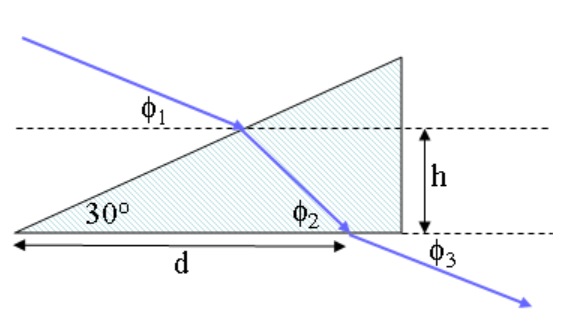# Problem: Red light is incident in air on a 30- 60- 90(degree) prism as shown. The incident beam is directed at an angle of ϕ1 = 41.3° and ϕ2 = 46.6° with respect to the horizontal and enters the prism at a height h = 17 cm above the base. The beam leaves the prism to the air at a distance d = 45.5 along the base as shown. The refractive index of the glass is 1.39.What is ϕ3, the angle the transmitted beam makes with the horizontal axis?

###### FREE Expert Solution

Snell's law:

$\overline{){{\mathbf{\eta }}}_{{\mathbf{1}}}{\mathbf{s}}{\mathbf{i}}{\mathbf{n}}{{\mathbf{\theta }}}_{{\mathbf{1}}}{\mathbf{=}}{{\mathbf{\eta }}}_{{\mathbf{2}}}{\mathbf{s}}{\mathbf{i}}{\mathbf{n}}{{\mathbf{\theta }}}_{{\mathbf{2}}}}$

η1 = ηglass = 1.39

η2 = ηair = 1###### Problem Details

Red light is incident in air on a 30- 60- 90(degree) prism as shown. The incident beam is directed at an angle of ϕ1 = 41.3° and ϕ2 = 46.6° with respect to the horizontal and enters the prism at a height h = 17 cm above the base. The beam leaves the prism to the air at a distance d = 45.5 along the base as shown. The refractive index of the glass is 1.39.What is ϕ3, the angle the transmitted beam makes with the horizontal axis?